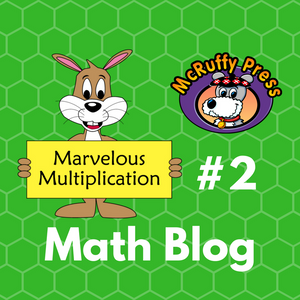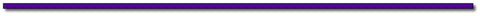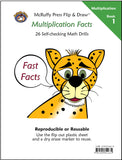# Marvelous Multiplication #2Marvelous Multiplication Trick #2: A Multiplication Challenge Using Easy Squares.

If you haven’t already, check out our previous three blogs:

Quick Math: Find the Squares of Numbers That End With 5

Quick Math: Squaring Numbers 51 to 59

In the previous post (Marvelous Multiplication #1) we generated and easily solved problems based on what we termed “easy squares” of two-digit numbers. This included the techniques learned in the two Quick Math blogs and squares of multiples of ten (10, 20, 30, 40…). We generated multipliers by adding and subtracting 1 to and from the easy squares.

In this post, we’ll expand the number of multiplication problems that can be generated and suggest a math challenge game.

The Trick:

Generate 2 two-digit multipliers around an easy square by adding and subtracting the same number to and from the easy square. In the last post, we subtracted 1, but the trick works with adding and subtracting any number. Let’s use the easy square of 30 for an example and add and subtract 2 for the multipliers. We’ll call the number 2 the “distance” between either multiplier and the easy square.

30 -2 = 28 and 30 + 2 = 32 Our multipliers will be 28 and 32.

To quickly find the product of 28 x 32 find the product of the easy square (302 = 900) and subtract the square of the distance. (22 = 4)

28 x 32 = 900 – 4 = 896

Let’s try it with a “distance” of 3:

30 – 3 = 27 and 30 + 3 = 33 Our multipliers will be 27 and 33.

27 x 33 = 900 – 9 = 891

Teaching Sheet 1 provides a form children can use to practice stepping through the process. Chose an easy square and write the digits in the boxes. Choose a “distance” number. Add and subtract the “distance” to generate multipliers for the problem.

Write the product of the easy square on the bottom row and the distance square. Subtract the distance squared to find the answer.

Challenge Game:

Given a two-digit number, generate a problem around an Easy Square and use the math tricks to solve the problem. The ultimate goal would be doing all the steps using mental math, but teaching sheets 2 and 3 present the steps involved in solving the problems to practice thinking through the steps.

For example, try the number 43.

You can make an easy square based problem around the Easy Square of 45. The distance between 43 and 45 is 2. (45 – 43 = 2) The second multiplier will be 47 (45 + 2 = 47). The problem generated will be 43 x 47.

The Easy Square of 45 is 2025. The Distance of 2 squared is 4.

2025 – 4 = 2021 so 43 x 47 = 2021

You could also make an easy square based problem around the Easy Square of 40. The distance between 43 and 40 is 3. (43 – 40 = 3) The second multiplier will be 37 (40 - 3 = 37). The problem generated will be 43 x 37.

Easy Square is 402 = 1600 and the Distance squared is 32 = 9

43 x 37 = 1600 – 9 = 1591

You could also make an easy square based problem around the Easy Square of 53. The distance between 43 and 53 is 10. The second multiplier will be 63. The problem generated will be 43 x 63.

The Easy Square is 532 = 2809 and the Distance squared is 102 = 100

43 x 63 = 2809 – 100 = 2709

Sheet 2 shows all the steps. Start with a random two-digit number and write it on the top line of the “Generate a Problem” section. Choose a nearby easy square. Write the number that is to be added or subtracted to equal the easy square. Next, write the easy square. Generate the second multiplier by doing the opposite operation to the Easy Square. Write the second multiplier in the boxes.

On the bottom row write the products of the squares of the Easy Square and Distance. Subtract for the answer to the generated multiplication problem.

Sheet 3 visually simplifies the process. The first section is used to write the multiplication problem starting with any given two-digit number. The ES boxes are for the Easy Square. The D boxes are for the Distance. Most of the time it will be a single digit distance, but it can be any number of digits. The sheet is set up for a distance with a maximum of two-digits. The far right boxes are for the squares of the Easy Square and Distance.

Encourage children to skip any boxes they can do mentally. For example, if they can write the product of the Easy Square directly in the far right boxes, skip the center box. If the distance and distance square are smaller numbers and easy to remember those boxes can be skipped.

Why it Works:

Let’s build an algebra problem. We’ll represent the Easy Square with the letter a and the “distance” with the letter b. We generated the multipliers by adding and subtracting the “distance” to and from an Easy Square.

(a – b)(a + b) = a2 + ab – ab – b2 =  a2 – b2

This is true for any “distance” even when it makes numbers negative and multipliers have more than two digits. For example, with the number 30 we can make the “distance” 200.

The multipliers are 230 and -170

Easy Square is 302 = 900 and The distance squared is 2002 = 40,000

230 x (-170) = 900 - 40,000 = -39,100## Thanks for Visting Savings:

Use the code MarMult2 to save \$3.00 off every Color Math Curriculum Package. See eligible products here.

Offer Expires July 30, 2017Subscribe to this blog's RSS feed using http://feeds.feedburner.com/McruffyPress-McruffyMathBlog## McRuffy Press offers complete math curriculum by grade level, math activity books, manipulatives, games and more! Find more McRuffy Math products here.

Improve multiplication facts skills with our Multiplication Fast Fact books:Multiplication Facts (Book 1) Drills factors from 1 to 10 in our self-checking, non-consumable format that allows students to practice until they have mastered basic facts.Multiplication Practice (Book 2) Drills factors from 1 to 10 in our self-checking, non-consumable format that allows students to practice until they have mastered basic facts.